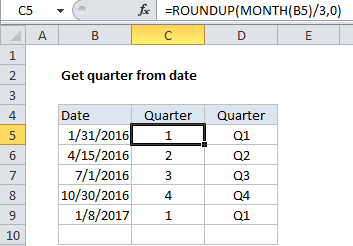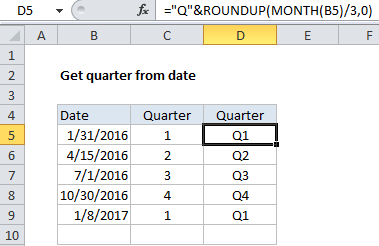# How to calculate quarter from date in Excel

If you want to calculate the quarter (i.e. 1,2,3,4) from a date, you can use the ROUNDUP function together with MONTH.

## Formula

`=ROUNDUP(MONTH(date)/3,0)`## Explanation

In the example shown, the formula in cell C5 is:

`=ROUNDUP(MONTH(B5)/3,0)`

### How this formula works

In this case, the formula first extracts the month as a number between 1-12, then divides this number by 3. The result is then rounded up to the nearest whole number using the ROUNDUP function.

Also See:   ROUNDUP function: Description, Usage, Syntax, Examples and Explanation

The ROUNDUP function works like the ROUND function, except that ROUNDUP will always round numbers 1-9 upwards to a given number of digits.

### Adding a Q

If you want the quarter number to include a “Q” you can concatenate. The formula in D5 is:

`="Q"&ROUNDUP(MONTH(B5)/3,0)`

The result is the letter “Q” prepended to the quarter number:

Also See:   Round numbers in Excel